Fit Analyses

# Nonparametric Smoothers

For a simple regression model with one or two explanatory variables,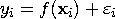a smoother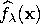is a function that summarizes the trend of Y as a function of X. It can enhance the visual perception of either a Y-by-X scatter plot or a rotating plot. The smoothing parameter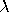controls the smoothness of the estimate.

With one explanatory variable in the model,is called a scatter plot smoother. SAS/INSIGHT software provides nonparametric curve estimates from smoothing spline, kernel, loess (nearest neighbors local polynomial), and fixed bandwidth local polynomial smoothers.

For smoothing spline, kernel, and fixed bandwidth local polynomial smoothers, SAS/INSIGHT software derives the smoothing parameterfrom a constant c that is independent of the units of X. For a loess smoother, the smoothing parameteris a positive constant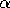.

With two explanatory variables in the model,is called a surface smoother. SAS/INSIGHT software provides nonparametric surface estimates from thin-plate smoothing spline and kernel smoothers. The explanatory variables are scaled by their corresponding sample interquartile ranges. The smoothing parameteris derived from a constant c and both are independent of the units of X.

Similar to parametric regression, the R2 value for an estimate is calculated as
You can use the following methods to choose thevalue:

DF
uses thevalue that makes the resulting smoothing estimate have the specified degrees of freedom (df).

GCV
uses thevalue that minimizes the generalized cross validation (GCV) mean squared error.

C Value
uses thevalue derived from the specified c value for nonparametric smoothers other than the loess smoother.

Alpha
uses the specifiedvalue for the loess estimator.

If you specify a DF value for a smoother, an iterative procedure is used to find the estimate with the specified df. You can choose a convergence criterion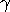based on either the relative difference or the absolute difference. A smoother satisfying the following conditions is then created: